fft变换 图像处理_图像fft变换 - CSDN
• 傅立叶变换以高等数学（微积分）中的傅立叶级数为基础发展而来，它是信号处理（特别是图像处理）中非常重要的一种时频变换手段，具有重要应用。在图像编码、压缩、降噪、数字水印方面都有重要意义。此外，快速傅立叶...
欢迎关注我的博客专栏“图像处理中的数学原理详解”

全文目录请见  图像处理中的数学原理详解（总纲）

http://blog.csdn.net/baimafujinji/article/details/48467225

傅立叶变换以高等数学（微积分）中的傅立叶级数为基础发展而来，它是信号处理（特别是图像处理）中非常重要的一种时频变换手段，具有重要应用。在图像编码、压缩、降噪、数字水印方面都有重要意义。此外，快速傅立叶变换算法还位列20世纪十大算法之列，它是“动态规划”策略在算法设计中的杰出代表。本文将详细介绍图像中的傅立叶变换及其快速算法。对于傅立叶变换的数学原理还不是很理解的同学，建议参考本系列前面已经发布的傅立叶级数相关内容，争取彻底搞懂相关数学原理。一知半解、不求甚解，都是自欺欺人的表现。

6.1.2   数字图像的傅立叶变换
为了在科学计算和数字信号处理等领域使用计算机进行傅立叶变换，必须将函数f(t)定义在离散点而非连续域内，且须满足有限性或周期性条件。这种情况下，使用离散傅立叶变换。将连续函数f(t)等间隔采样就得到一个离散序列f(x)，假设采样N次，则这个离散序列可以表示为{f(0),f(1),f(2),...,f(N-1)}。如果令x为离散实变量，u为离散频率变量，则一维离散傅立叶变换的正变换定义为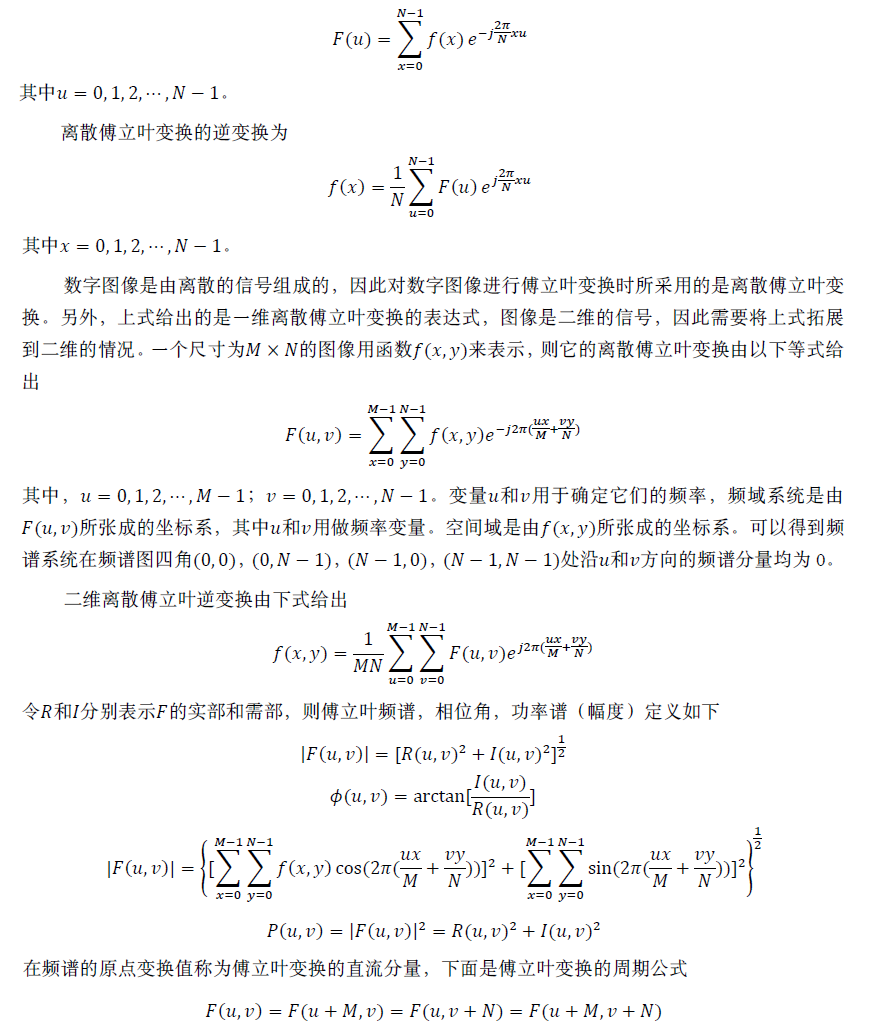图像的频率是表征图像中灰度变化剧烈程度的指标，是灰度在平面空间上的梯度。从傅立叶频谱图上看到的明暗不一的亮点，实际上图像上某一点与邻域点差异的强弱，即梯度的大小，也即该点的频率的大小（可以这么理解，图像中的低频部分指低梯度的点，高频部分相反）。通常，梯度大则该点的亮度强，否则该点亮度弱。这样通过观察傅立叶变换后的频谱图，也叫功率图。在功率图中我们可以看出图像的能量分布，如果频谱图中暗的点数更多，那么实际图像是比较柔和的（因为各点与邻域差异都不大，梯度相对较小），反之，若频谱图中亮的点数多，那么实际图像一定是尖锐的，边界分明且边界两边像素差异较大的。对频谱移频到原点以后，可以看出图像的频率分布是以原点为圆心，对称分布的。变换最慢的频率成分（u = v = 0）对应一幅图像的平均灰度级。当从变换的原点移开时，低频对应着图像的慢变换分量，较高的频率开始对应图像中变化越来越快的灰度级。这些是物体的边缘和由灰度级的突发改变（如噪声）标志的图像成分。通常在进行傅立叶变换之前用(-1)^(x+y)乘以输入的图像函数，这样便可将傅立叶变换的原点(0,0)移到(M/2,N/2)上。

6.1.3  快速傅立叶变换的算法

离散傅立叶变换（DFT）已经成为数字信号处理和图像处理的一种重要手段，但是DFT的计算量太大，速度太慢，这令其实用性大打折扣。1965年，Cooley和Tukey提出了一种快速傅立叶变换算法（Fast Fourier Transform，FFT），极大地提供了傅立叶变换的速度。正是FFT的出现，才使得傅立叶变换得以广泛地应用。

FFT并不是一种新的变换，它只是傅立叶变换算法实现过程的一种改进。FFT中比较常用的是蝶形算法。蝶形算法主要是利用傅立叶变换的可分性、对称性和周期性来简化DFT的运算量。下面就来介绍一下蝶形算法的基本思想。

由于二维离散傅立叶变换具有可分离性， 即它可由两次一维离散傅立叶变换计算得到，因此仅研究一维离散傅立叶变换的快速算法即可。一维离散傅立叶变换的公式为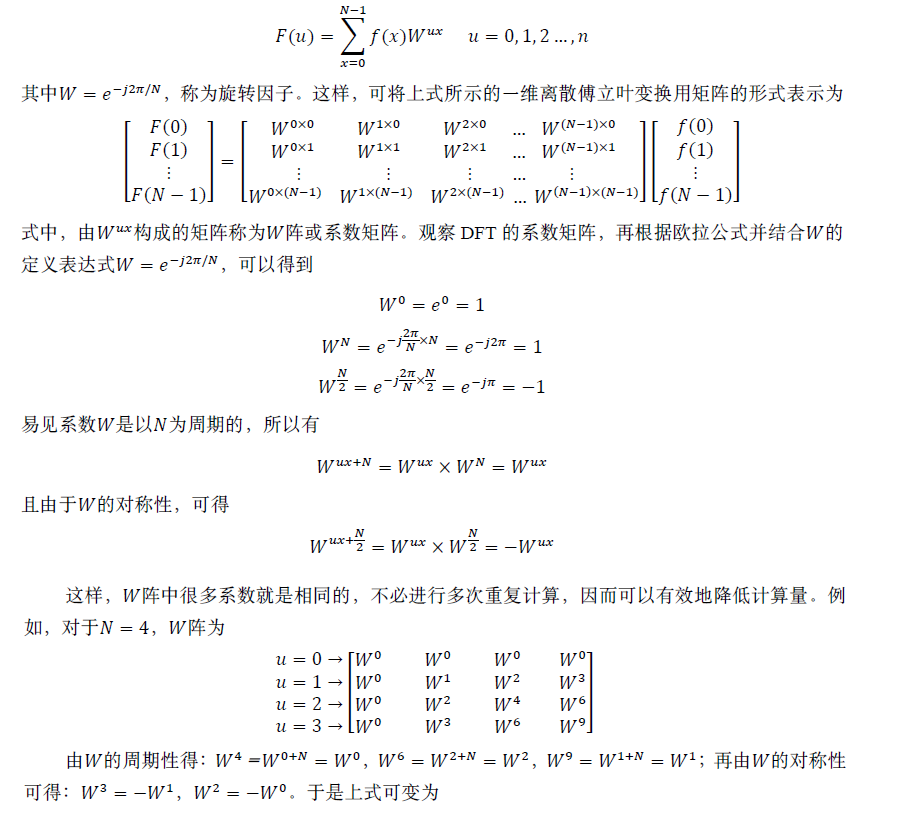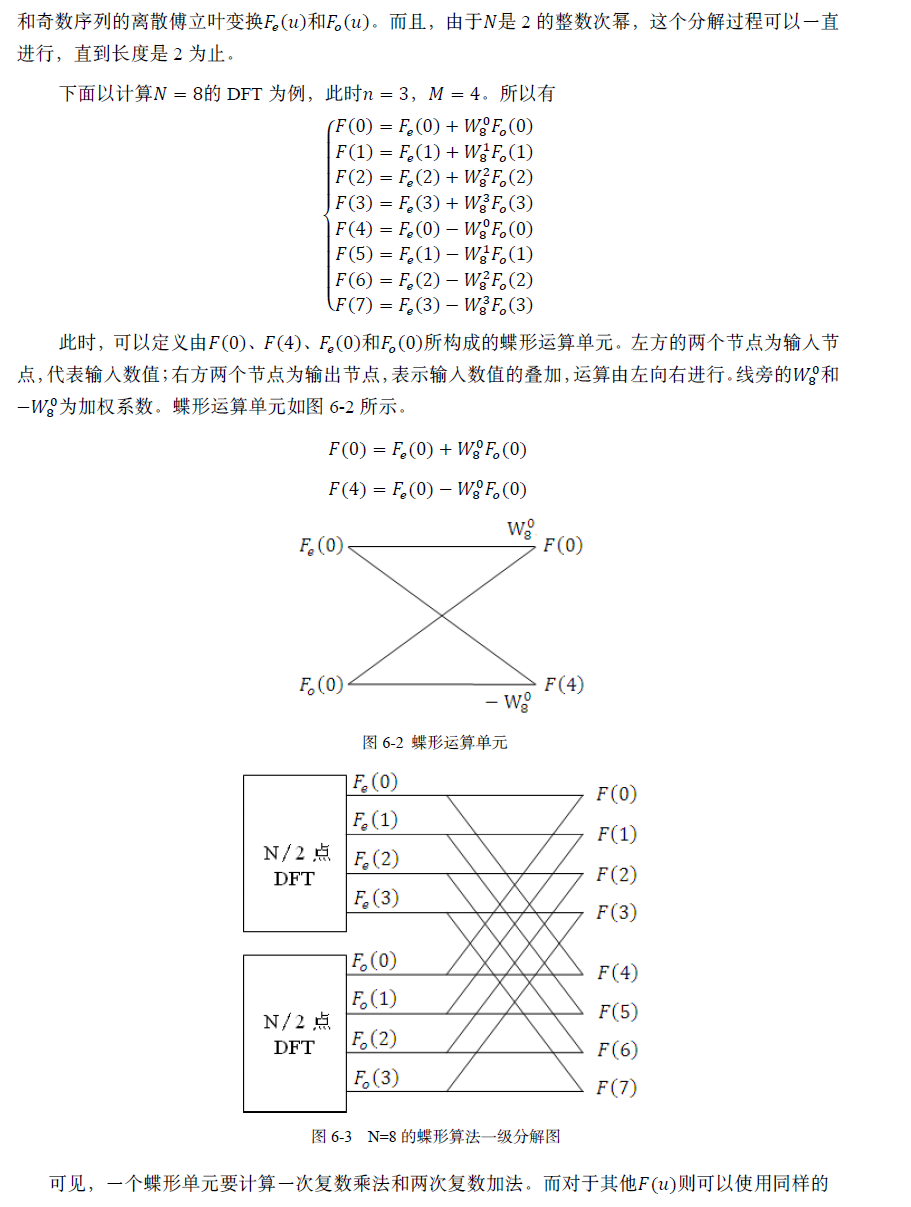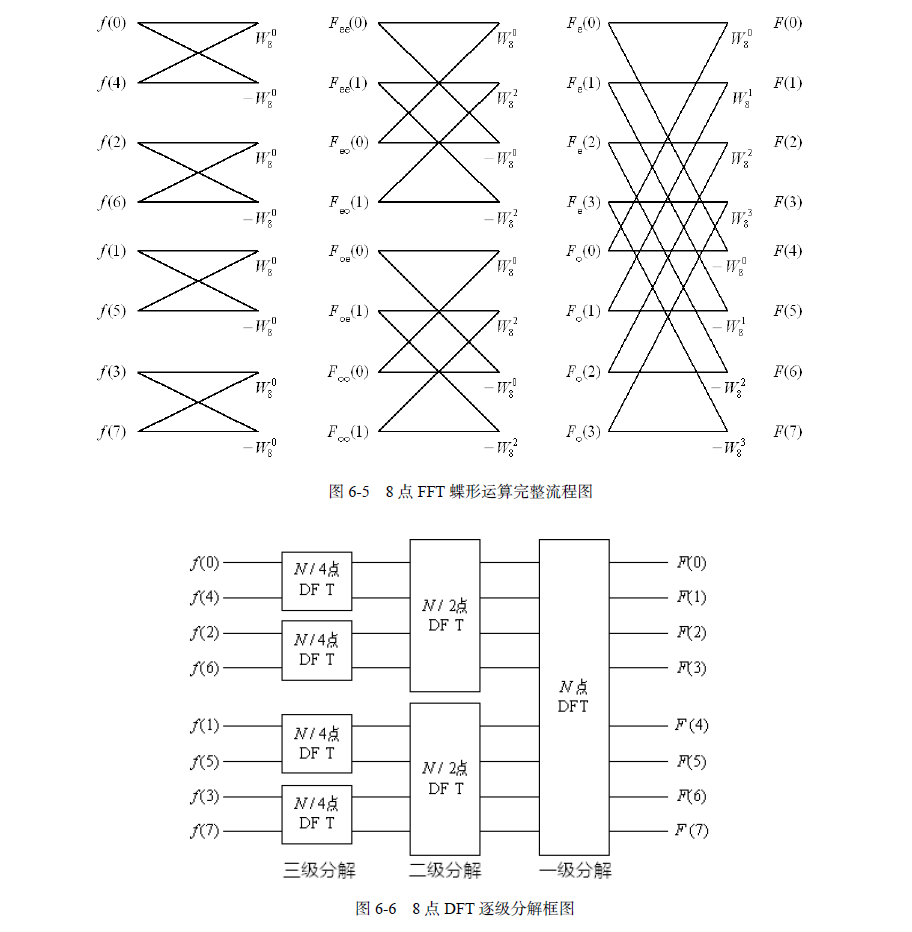上述FFT是将f(x)序列按x的奇偶进行分组计算的，称之为时间抽选FFT。如果将频域序列的F(u)按u的奇偶进行分组计算，也可实现快速傅立叶计算，这称为频率抽选FFT。

通过对图6-6的观察可以发现，蝶形算法的频率域是按照正常顺序排列的，而空间域是按照一种叫做“码位倒序”的方式排列的。这个倒序的过程可以采用下面的方法来实现：将十进制的数转化成二进制，然后将二进制的序列倒序重排，最后再把颠倒顺序后的二进制数转换成十进制数。倒序重排的程序是一段经典程序，它以巧妙的构思、简单的语句用完成了倒序重排的功能。表6-1给出了倒序重排的示例。我的“图像处理中的数学原理”专栏中之系列文章已经以《图像处理中的数学修炼》为名结集出版（清华大学出版社）。该书详细介绍图像处理中的数学原理，为你打开一道通往图像世界的数学之门，详细内容及目录请见 http://blog.csdn.net/baimafujinji/article/details/48467225


展开全文算法 图像处理 傅立叶变换
• 一，读懂傅里叶变换 1，关于两大域：时域与频域 （1），频域（frequency domain）是指在对函数或信号进行分析时， 分析其和频率有关部份，而不是和时间有关的部份，和时域一词相对。 （2），时域是描述数学函数或...
以下部分文字资料整合于网络，本文仅供自己学习用！

这是一幅很绝的一维傅里叶变换动态图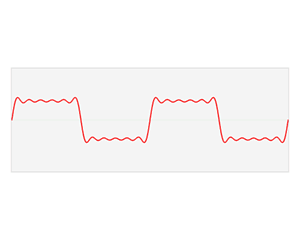一，读懂傅里叶变换
一个信号能表示成傅里叶级数的形式是有条件的，首先它必须是周期信号，第二必须是满足狄里赫利条件的周期信号。
1，关于两大域：时域与频域
（1），频域（frequency domain）是指在对函数或信号进行分析时，

分析其和频率有关部份，而不是和时间有关的部份，和时域一词相对。
（2），时域是描述数学函数或物理信号对时间的关系。
例如一个信号的时域波形可以表达信号随着时间的变化。
若考虑离散时间，时域中的函数或信号，在各个离散时间点的数值均为已知。
若考虑连续时间，则函数或信号在任意时间的数值均为已知。在研究时域的信号时，常会用示波器将信号转换为其时域的波形。
（3），两者相互间的变换
时域（信号对时间的函数）和频域（信号对频率的函数）的变换在数学上是通过积分变换实现。
对周期信号可以直接使用傅立叶变换，对非周期信号则要进行周期扩展，使用拉普拉斯变换。

2，傅立叶变换
（1），什么是傅里叶变换？也称作傅立叶变换，表示能将满足一定条件的某个函数表示成三角函数（正弦和/或余弦函数）或者它们的积分的线性组合。在不同的研究领域，傅立叶变换具有多种不同的变体形式，如连续傅立叶变换和离散傅立叶变换。最初傅立叶分析是作为热过程的解析分析的工具被提出的。傅里叶变换是一种分析信号的方法，它可分析信号的成分，也可用这些成分合成信号。许多波形可作为信号的成分，比如正弦波、方波、锯齿波等，傅里叶变换用正弦波作为信号的成分。傅里叶变换的实质是将一个信号分离为无穷多多正弦/复指数信号的加成，也就是说，把信号变成正弦信号相加的形式——既然是无穷多个信号相加，那对于非周期信号来说，每个信号的加权应该都是零——但有密度上的差别，你可以对比概率论中的概率密度来思考一下——落到每一个点的概率都是无限小，但这些无限小是有差别的所以，傅里叶变换之后，横坐标即为分离出的正弦信号的频率，纵坐标对应的是加权密度
（2），傅里叶变换有什么用呢？举例说明：傅里叶变换可以将一个时域信号转换成在不同频率下对应的振幅及相位，其频谱就是时域信号在频域下的表现，而反傅里叶变换可以将频谱再转换回时域的信号。最简单最直接的应用就是时频域转换，比如在移动通信的LTE系统中，要把接收的信号从时域变成频域，就需要使用FFT。又例如对一个采集到的声音做傅立叶变化就能分出好几个频率的信号。比如南非世界杯时，南非人吹的呜呜主拉的声音太吵了，那么对现场的音频做傅立叶变化（当然是对声音的数据做），会得到一个展开式，然后找出呜呜主拉的特征频率，去掉展开式中的那个频率的sin函数，再还原数据，就得到了没有呜呜主拉的嗡嗡声的现场声音。而对图片的数据做傅立叶，然后增大高频信号的系数就可以提高图像的对比度。同样，相机自动对焦就是通过找图像的高频分量最大的时候，就是对好了。

3，快速傅里叶变换
（1），什么是快速傅里叶变换？
计算离散傅里叶变换的一种快速算法，简称FFT。快速傅里叶变换是1965年由J.W.库利和T.W.图基提出的。采用这种算法能使计算机计算离散傅里叶变换所需要的乘法次数大为减少，特别是被变换的抽样点数N越多，FFT算法计算量的节省就越显著。

（2），快速傅里叶变换有什么用？
函数或信号可以透过一对数学的运算子在时域及频域之间转换。和傅里叶变换作用一样！

（3），为什么要提出快速傅里叶变换？
人们想让计算机能处理信号 但由于信号都是连续的、无限的，计算机不能处理，于是就有了傅里叶级数、傅里叶变换，将信号由时域变到频域，把一个信号变为有很多个不同频率不同幅度的正弦信号组成，这样计算机就能处理了，但又由于傅里叶变换中要用到卷积计算，计算量很大，计算机也算不过来，于是就有了快速傅里叶变换，大大降低了运算量，使得让计算机处理信号成为可能。快速傅里叶变换是傅里叶变换的快速算法而已，主要是能减少运算量和存储开销，对于硬件实现特别有利。
4，图像中傅立叶变换的物理意义
（1），图像的频率是表征图像中灰度变化剧烈程度的指标，是灰度在平面空间上的梯度。如：大面积的沙漠在图像中是一片灰度变化缓慢的区域，对应的频率值很低；而对于地表属性变换剧烈的边缘区域在图像中是一片灰度变化剧烈的区域，对应的频率值较高。傅立叶变换在实际中有非常明显的物理意义，设f是一个能量有限的模拟信号，则其傅立叶变换就表示f的谱。从纯粹的数学意义上看，傅立叶变换是将一个函数转换为一系列周期函数来处理的。从物理效果看，傅立叶变换是将图像从空间域转换到频率域，其逆变换是将图像从频率域转换到空间域。换句话说，傅立叶变换的物理意义是将图像的灰度分布函数变换为图像的频率分布函数，傅立叶逆变换是将图像的频率分布函数变换为灰度分布函数

（2），傅立叶变换以前，图像（未压缩的位图）是由对在连续空间（现实空间）上的采样得到一系列点的集合，我们习惯用一个二维矩阵表示空间上各点，则图像可由z=f(x,y)来表示。由于空间是三维的，图像是二维的，因此空间中物体在另一个维度上的关系就由梯度来表示，这样我们可以通过观察图像得知物体在三维空间中的对应关系。为什么要提梯度？因为实际上对图像进行二维傅立叶变换得到频谱图，就是图像梯度的分布图，当然频谱图上的各点与图像上各点并不存在一一对应的关系，即使在不移频的情况下也是没有。傅立叶频谱图上我们看到的明暗不一的亮点，实际上图像上某一点与邻域点差异的强弱，即梯度的大小，也即该点的频率的大小（可以这么理解，图像中的低频部分指低梯度的点，高频部分相反）。一般来讲，梯度大则该点的亮度强，否则该点亮度弱。这样通过观察傅立叶变换后的频谱图，也叫功率图，我们首先就可以看出，图像的能量分布，如果频谱图中暗的点数更多，那么实际图像是比较柔和的（因为各点与邻域差异都不大，梯度相对较小），反之，如果频谱图中亮的点数多，那么实际图像一定是尖锐的，边界分明且边界两边像素差异较大的。对频谱移频到原点以后，可以看出图像的频率分布是以原点为圆心，对称分布的。将频谱移频到圆心除了可以清晰地看出图像频率分布以外，还有一个好处，它可以分离出有周期性规律的干扰信号，比如正弦干扰，一副带有正弦干扰，移频到原点的频谱图上可以看出除了中心以外还存在以某一点为中心，对称分布的亮点集合，这个集合就是干扰噪音产生的，这时可以很直观的通过在该位置放置带阻滤波器消除干扰

二，理性认识傅里叶变换
1，二维傅里叶fft2对简单矩阵的操作
（可以把s想象成一幅图像，傅里叶变换过程如下）

s=magic(2);%magic矩阵有一个神奇的特点：每行或者每列加起来都相等
f=fft2(s);
a=abs(f)

a =

10     0
2     4

>> f

f =

10     0
-2    -4

>> s

s =

1     3
4     2

%3*3的矩阵的操作结果
s=magic(3);
f=fft2(s);
a=abs(f)

a =

45.0000         0         0
0.0000   15.5885    5.1962
0.0000    5.1962   15.5885

>> f

f =

45.0000                  0                  0
0 + 0.0000i  13.5000 + 7.7942i   0.0000 - 5.1962i
0 - 0.0000i   0.0000 + 5.1962i  13.5000 - 7.7942i

>> s

s =

8     1     6
3     5     7
4     9     2

%4*4的矩阵操作结果
s=magic(4);%magic矩阵有一个神奇的特点：每行或者每列加起来都相等
f=fft2(s);
a=abs(f)

a =

136.0000         0         0         0
0   20.0000   11.3137   12.0000
0   45.2548         0   45.2548
0   12.0000   11.3137   20.0000

>> f

f =

1.0e+02 *

1.3600                  0                  0                  0
0             0.2000             0.0800 + 0.0800i        0 - 0.1200i
0             0.3200 + 0.3200i        0             0.3200 - 0.3200i
0                  0 + 0.1200i   0.0800 - 0.0800i   0.2000

>> s

s =

16     2     3    13
5    11    10     8
9     7     6    12
4    14    15     12，二维快速傅里叶fft2对灰度图的操作

<span style="font-size:12px;">clear all;
close all;
J=rgb2gray(I);%将图像转换为灰度图
K=fft2(J);%对图像进行二维快速傅里叶变换
K=fftshift(K);%将频谱转移到中心，其实就是在傅里叶变换时乘以了某个因子
L=abs(K/256);%取模
%显示图片
figure;
subplot(131);
imshow(I);title('原图像')
subplot(132);
imshow(J);title('被转换为灰度图后')
subplot(133);
imshow(uint8(L));title('二维傅里叶变换后的频谱图')</span>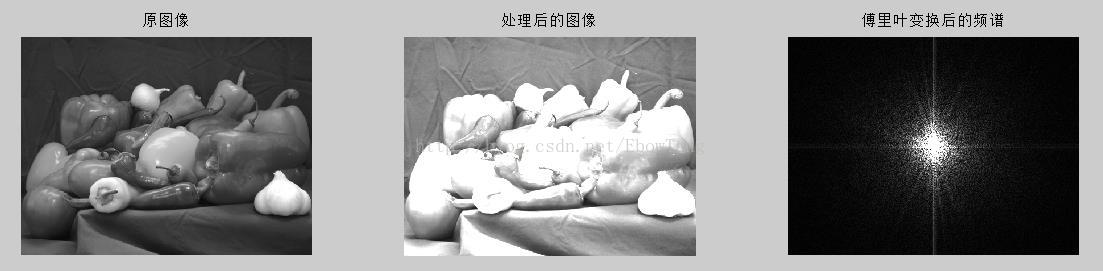clear all; close all;
J=rgb2gray(I);%转换为灰度图
J=imrotate(J,45,'bilinear');	%将图像旋转45度角
K=fft2(J);%对图像进行二维傅里叶比变换
K=fftshift(K);%转移频谱中心
L=abs(K/256);%取模
figure;
subplot(121);
imshow(J);title('原图像被旋转45度角')
subplot(122);
imshow(uint8(L));title('傅里叶变换后的图像')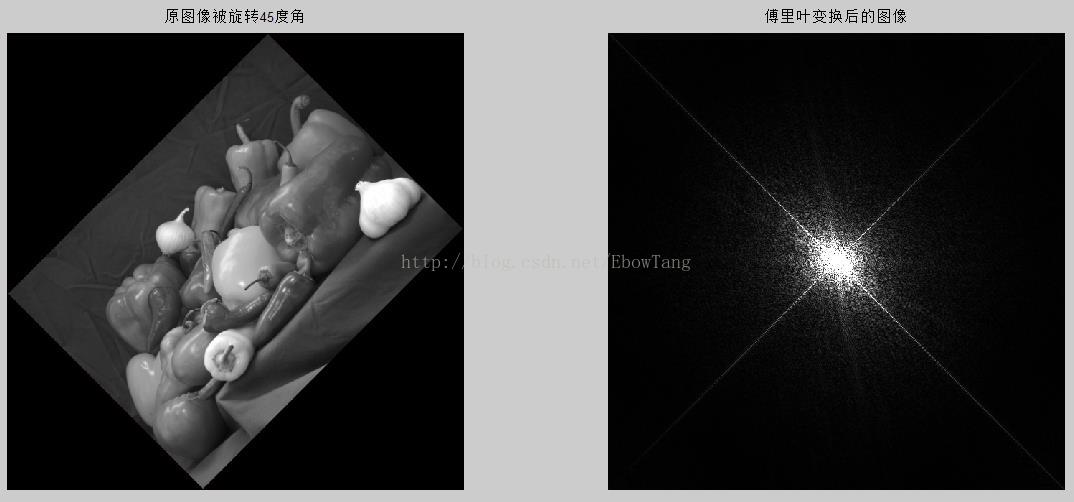clear all; close all;
J=rgb2gray(I);
J=imnoise(J, 'gaussian', 0, 0.01);%加入高斯噪声
K=fft2(J);
K=fftshift(K);
L=abs(K/256);
figure;
subplot(131);
imshow(I);title('原图像')
subplot(132);
imshow(J);title('高斯噪声污染')
subplot(133);
imshow(uint8(L));title('污染后进行福利叶变换')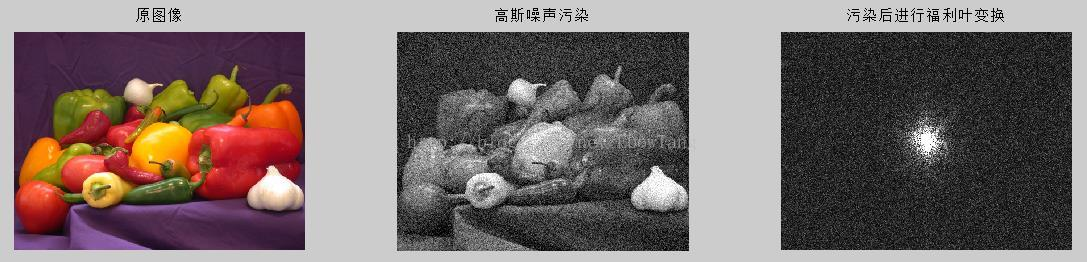clear all; close all;
J=rgb2gray(I);
K=fft2(J);%傅里叶变换
L=fftshift(K);%转移频谱中心
L=abs(L/256);%取模
M=ifft2(K);%二维傅里叶反变换
figure;
subplot(131);
imshow(J);title('原灰度图像')
subplot(132);
imshow(uint8(L));title('傅里叶变换后的图像')
subplot(133);
imshow(uint8(M));title('反变换后的图像')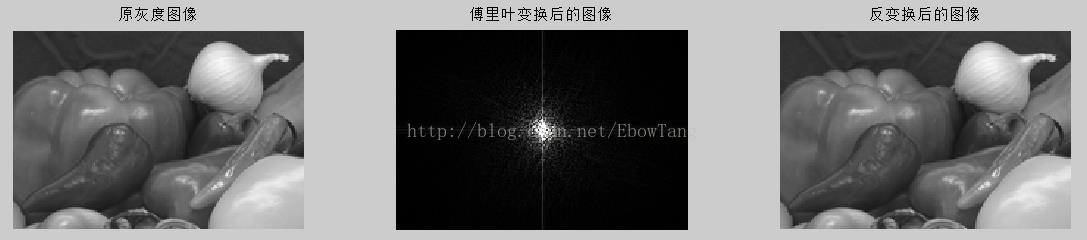参考资源：
【1】http://blog.csdn.net/struggle_for_m/article/details/51207370
【2】动态图来源于“维基百科”
【3】https://zhuanlan.zhihu.com/p/19763358?columnSlug=wille


展开全文图像处理 傅里叶变换
• 使用Fourier变换把图像从空间域变换到频域，在频域内做相应增强处理，再从频域变换到空间域得到处理后的图像,我们这里主要学习Fourier变换和FFT变换的算法.
注：本系列来自于图像处理课程实验，用Matlab实现最基本的图像处理算法

1.Fourier变换

（1）频域增强

除了在空间域内可以加工处理图像以外，我们还可以将图像变换到其他空间后进行处理，这些方法称为变换域方法，最常见的变换域是频域。

使用Fourier变换把图像从空间域变换到频域，在频域内做相应增强处理，再从频域变换到空间域得到处理后的图像。

我们这里主要学习Fourier变换和FFT变换的算法，没有学过通信原理，我对信号、时域分析也不是很清楚。

2.FFT算法

（1）离散Fourier变换，DFT

函数f(x)$f(x)$的DFT F(v)$F(v)$的计算式为：

F(v)=∑x=1Nf(x)e−i2πvxN,v=1,2,...,N
F(v)=\sum_{x=1}^N f(x) e^{\frac{-i2\pi vx}{N}},v=1,2,...,N

很显然求出所有的长度为N的信号的DFT需要N×O(N)=O(N2)$N\times O(N)=O(N^2)$的时间，比较慢，因此我们需要引入时间复杂度为O(NlogN)$O(N\log N)$的FFT算法。

（2）快速Fourier变换，FFT

快速傅立叶变换FFT是利用单位复数根的特殊性质（消去引理和折半引理，见《算法导论》（第3版中文版）P532详细论述），在O(NlogN)$O(N\log N)$时间内计算出DFT的一种快速算法。

FFT有两种基本实现方式：

递归FFT
迭代FFT，也叫FFT蝶式运算

递归FFT由于递归栈开销大且容量有限等缺陷（但理解容易），一般计算采取迭代FFT实现。

（3）迭代FFT

直接给出算法导论版本的迭代FFT算法：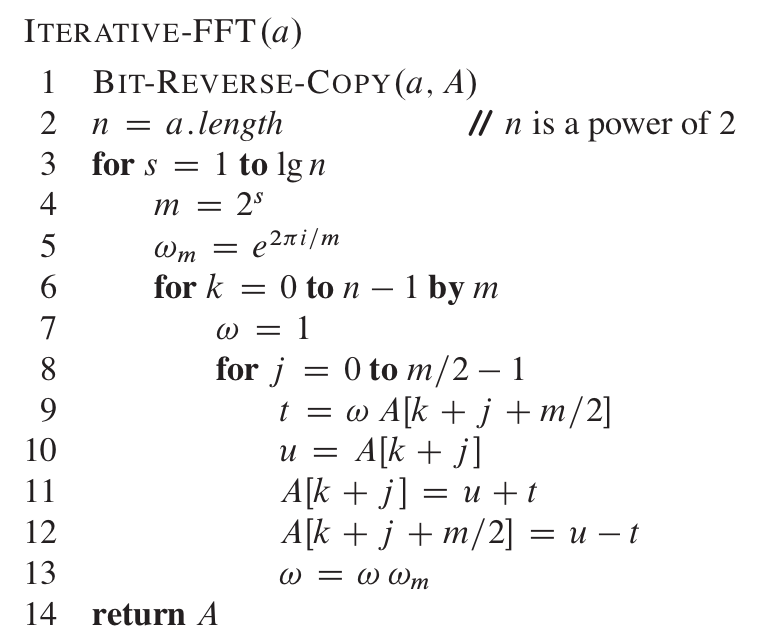其中求逆序数拷贝的函数为：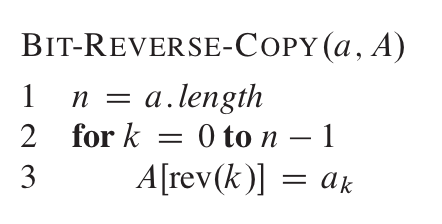FFT采用折半迭代的思想，因此速度能降低到O(NlogN)$O(N\log N)$。

（4）迭代FFTMatlab实现

Matlab有fft函数，我们也可以自己实现：

function [ fft_m ] = IterativeFFT( vec )
clear i;

n = length(vec);

fft_m = BitReverseCopy(vec);
for s = 1 : log2(n)
m = power(2, s);
wm = exp(- 2 * pi * i / m);

for k = 0 : m : n - 1
w = 1;
for j = 0 : m / 2 - 1
t = w * fft_m(k + j + m / 2 + 1);
u = fft_m(k + j + 1);
fft_m(k + j + 1) = u + t;
fft_m(k + j + m / 2 + 1) = u - t;
w = w * wm;
end
end
end
end

BitReverseCopy函数如下：

function [ copy ] = BitReverseCopy( vec )
n = length(vec);
copy = zeros(1, n);

bitn = log2(n);

for i = 0 : n - 1
revi = bin2dec(fliplr(dec2bin(i, bitn)));
copy(revi + 1) = vec(i + 1);
end
end

需要特别注意的是:

一般给出的FFT算法伪代码都是基于下标从零开始的数组，写在Matlab需要考虑映射关系
clear i是为了怕之前有变量i和复数记号i混淆，清楚Matlab workspace中的缓存
默认vec是double类型的！因为中间采用许多double类型运算

3.图像的二维Fourier变换

（1）二维DFT

二维DFT定义公式为：
F(u,v)=∑x=1N∑y=1Nf(x,y)e−i2π(ux+vy)N,u,v=1,2,...,N
F(u,v)=\sum_{x=1}^N \sum_{y=1}^N f(x,y) e^{\frac{-i2\pi (ux+vy)}{N}},u,v=1,2,...,N

计算一个频域点需要O(N2)$O(N^2)$的时间，那么整个二维频域计算需要O(N4)$O(N^4)$的时间，效率很低。

（2）将二维DFT分解为两个一维DFT

Fourier变换的变换核（公式中和f(x,y)$f(x,y)$无关的部分）具有对称的性质（详见《图像工程（上册）图像处理》P81），因此利用对称性可以将二维DFT分解为两个一维DFT：
先对二维矩阵的每一列做一维DFT：
F(x,v)=∑y=1Nf(x,y)e−i2πvyN,v=1,2,...,N
F(x,v)=\sum_{y=1}^N f(x,y) e^{\frac{-i2\pi vy}{N}},v=1,2,...,N

再对变换后的矩阵的每一行做一维DFT：

F(u,v)=∑x=1NF(x,v)e−i2πuxN,u=1,2,...,N
F(u,v)=\sum_{x=1}^N F(x,v) e^{\frac{-i2\pi ux}{N}},u=1,2,...,N

最后以2×N×O(N2)=O(N3)$2 \times N \times O(N^2)=O(N^3)$的时间完成二维傅立叶变换。

（3）用一维FFT实现二维FFT

同样的我们可以用两个一维FFT实现二维FFT，时间复杂度O(N2logN)$O(N^2 \log N)$：

function [ mfft2 ] = JCGuoFFT2( data )
h = size(data, 1);
w = size(data, 2);
mfft2 = data;

if power(2, log2(h)) ~= h || power(2, log2(w)) ~= w
disp('JCGuoFFT2 exit: h and w must be the power of 2!')
else
for i = 1 : h
mfft2(i, :) = IterativeFFT(mfft2(i, :));
end

for j = 1 : w
mfft2(:, j) = IterativeFFT(mfft2(:, j));
end
end
end


代码很简单，先做FFT行变换再做FFT列变换。之前忘记提到，我这里实现的都是长度必须是2的次方的Fourier变换，因此有时候会做一些长度规范检查。

（4）变换结果

经过JCGuoFFT2的二维傅立叶变换，我们可以得到复平面内各个点的复数值，那么显示在图像中需要先求出幅值：

pic1_fft = JCGuoFFT2(double(pic1));
pic1_fft_amp = abs(pic1_fft);

在对幅值做一次log变换得到较好的频域图像：

pic1_fft_amp_log = log(1 + pic1_fft_amp);

绘制结果如下图：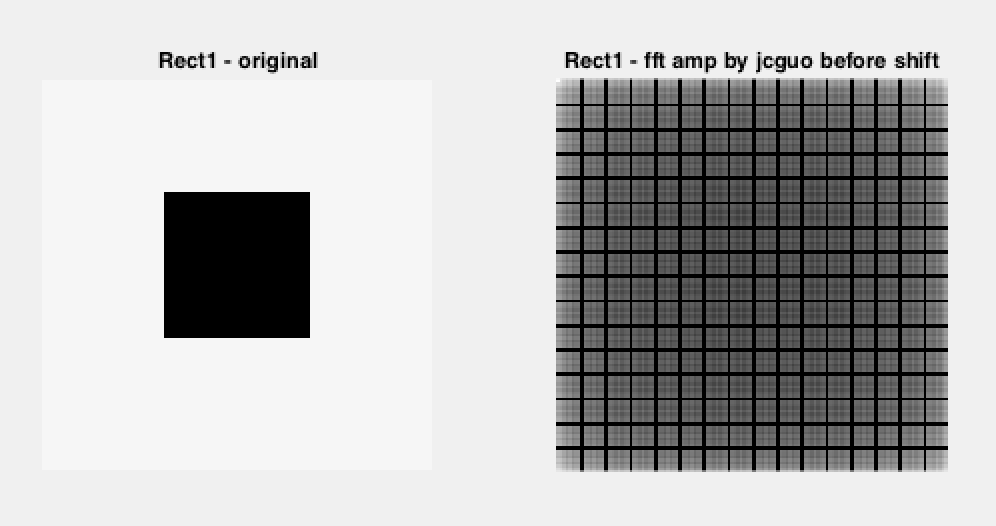（5）低频信号移到图像中心点

由于复数运算的周期特性，图像的Fourier变换在复平面上是完全对称的，可以想象平面是由无限多个上图（右）频域图像拼接而成的二维阵列。一般研究频域图像是把低频部分，也就是变换后的边缘部分移到图像中心点，Matlab提供fftshift函数完成平移。

平移的思路有两个

通过Fourier变换平移定理先把原始图像做变换再做FFT
先做FFT后再根据频域图像的对称性做对称变换

查阅Matlab文档发现它是采用第二种方法，对图像做以下子矩阵交换：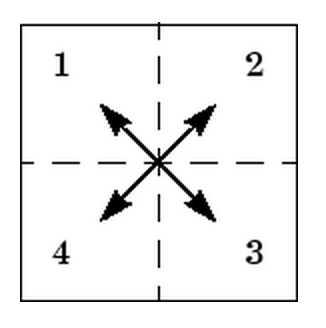那么我们可以很容易的写出自己的fftshift：

function [ after ] = FFTShift( before )
h = size(before, 1);
w = size(before, 2);

after = before;

if power(2, log2(h)) ~= h || power(2, log2(w)) ~= w
disp('FFTShift exit: h and w must be the power of 2!')
else
hh = h / 2;
hw = w / 2;
after(1 : hh, 1 : hw) = before(hh + 1 : h, hw + 1 : w);
after(1 : hh, hw + 1 : w) = before(hh + 1 : h, 1 : hw);
after(hh + 1 : h, 1 : hw) = before(1 : hh, hw + 1 : w);
after(hh + 1 : h, hw + 1 : w) = before(1 : hh, 1 : hw);
end
end


将低频部分平移到中心点后结果为：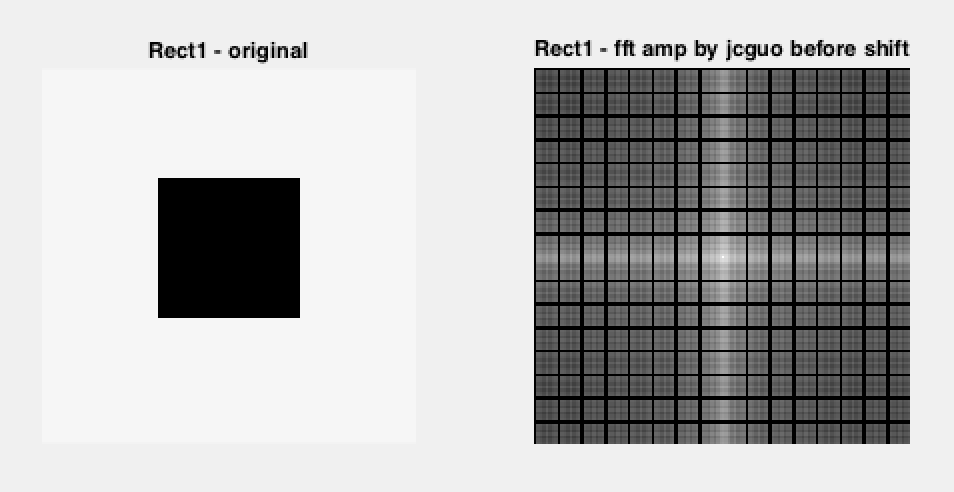4.图像的二维反Fourier变换

（1）一维逆DFT和一维逆FFT

一维离散傅立叶变换的逆变换是将e的指数部分变号，然后整体除以长度N（Fourier变换与逆变换关于符号、系数有很多种组合的定义，但他们都是等价且对称的。这里的定义配合Matlab做fft实际效果。）：

F(v)=1N∑x=1Nf(x)ei2πvxN,v=1,2,...,N
F(v)=\frac{1}{N} \sum_{x=1}^N f(x) e^{\frac{i2\pi vx}{N}},v=1,2,...,N

同样我们可以根据iDFT的定义推演iFFT的算法，其迭代版本的Matlab实现如下：

function [ ifft_m ] = IterativeIFFT( vec )
clear i;
n = length(vec);
ifft_m = BitReverseCopy(vec);
for s = 1 : log2(n)
m = power(2, s);
wm = exp(2 * pi * i / m);

for k = 0 : m : n - 1
w = 1;
for j = 0 : m / 2 - 1
t = w * ifft_m(k + j + m / 2 + 1);
u = ifft_m(k + j + 1);
ifft_m(k + j + 1) = u + t;
ifft_m(k + j + m / 2 + 1) = u - t;
w = w * wm;
end
end
end
ifft_m = ifft_m ./ n;
end


（2）二维逆FFT

二维逆FFT和二维FFT的思路一致，对所有行和列分别作一次iFFT即可：

function [ mifft2 ] = JCGuoIFFT2( data )
h = size(data, 1);
w = size(data, 2);
mifft2 = data;

if power(2, log2(h)) ~= h || power(2, log2(w)) ~= w
disp('JCGuoIFFT2 exit: h and w must be the power of 2!')
else
for i = 1 : h
mifft2(i, :) = IterativeIFFT(mifft2(i, :));
end

for j = 1 : w
mifft2(:, j) = IterativeIFFT(mifft2(:, j));
end
end
end


（3）逆FFT结果

对之前Rect1.bmp用JCGuoFFT2变换的到的Fourier变换（非shift和log之后、非仅幅度部分）作FFT2逆变换可以直接得到原图像：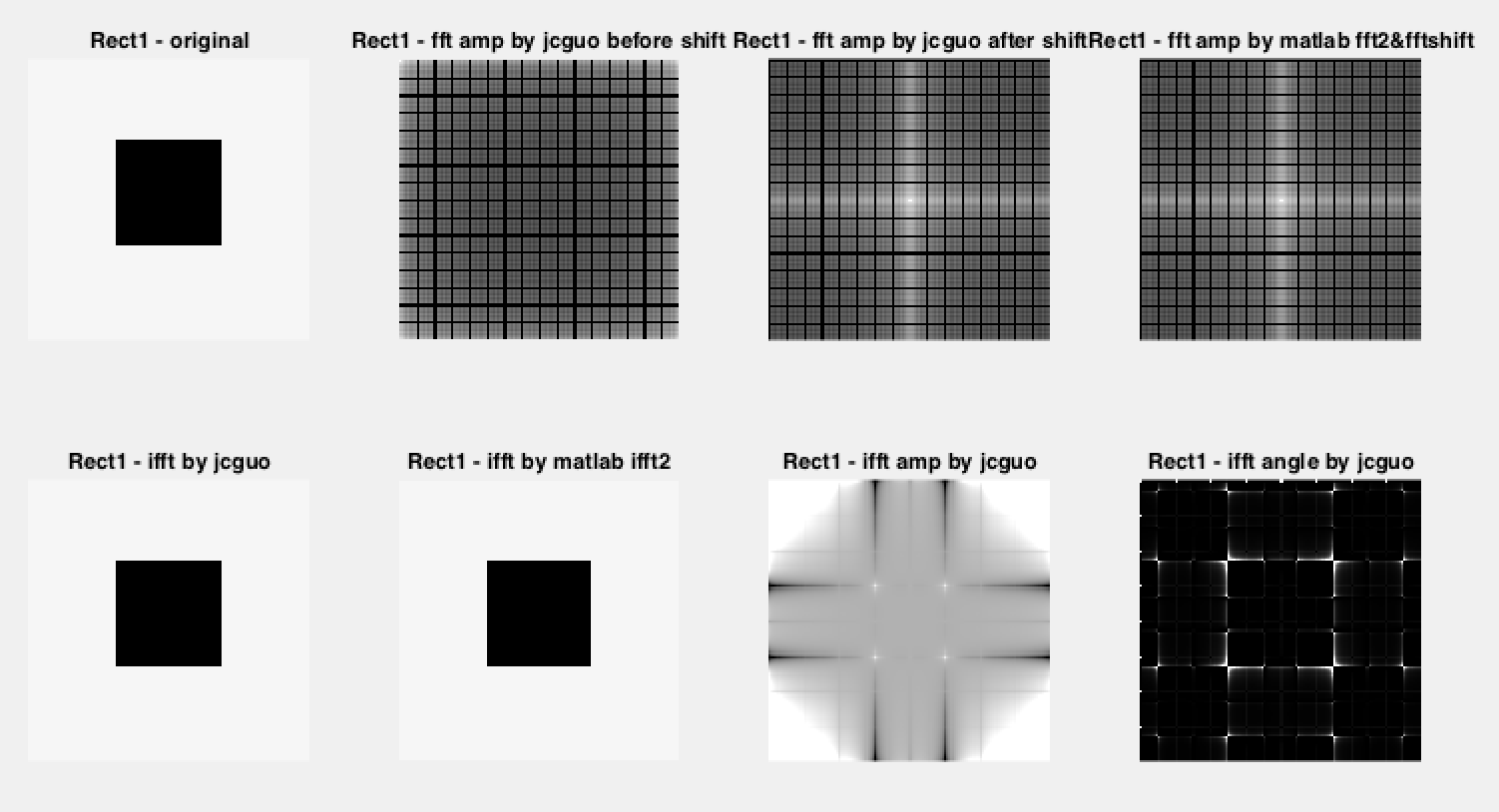这幅图有多个结果，可以看title知道每个结果的含义，图2-1是用JCGuoIFFT2做傅立叶反变换的结果，得到的图像和原图像、和Matlab ifft2反变换后的图像都是一致的。

5.幅频特性与相频特性

（1）对振幅和相位单独做逆FFT

如果我们只把图像Fourier变换的振幅部分和相位部分单独做二维逆FFT，可以直观的感受人眼对图像幅频特性和相频特性的敏感度。

复数z=a+ib$z=a+ib$的幅度/振幅定义为：

|z|=a2+b2‾‾‾‾‾‾‾√
|z|=\sqrt{a^2+b^2}

相位角和相位定义为：

ϕ(z)=arctanbaeiϕ(z)=eiarctanba
\phi(z)=\arctan\frac{b}{a}\\\\
e^{i\phi(z)}=e^{i\arctan\frac{b}{a}}

对相位反变换需要在乘以一个系数（我是调出来的，针对图像，肯定可以自动的做均衡化）：

pic2_fft_angle = angle(pic2_fft);
clear i;
tmp = 10000 * exp(i * pic2_fft_angle);
pic2_ifft_angle = uint8(JCGuoIFFT2(tmp));


对振幅和相位单独做逆FFT结果如下：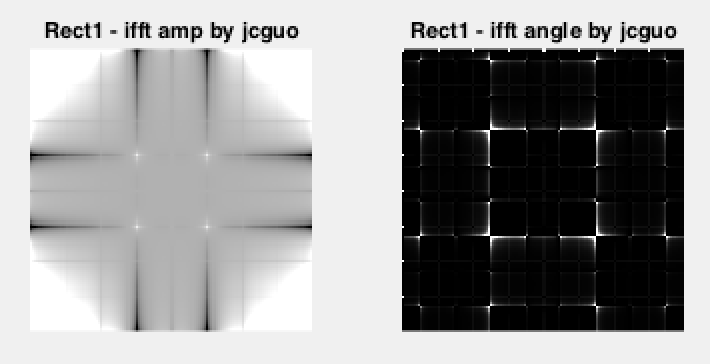（2）人眼敏感度

观察上图，幅频特性主要涵盖了图像颜色的分布，相频特性主要刻画了图像的边界信息。人眼对图像的相频特性更加敏感，看相频特性能够大概知道图像内容。

6.Fourier变换的旋转定理

（1）Fourier变换旋转定理

将f(x,y)旋转角度θ0，其Fourier变换F(u,v)也旋转角度θ0
将f(x,y)旋转角度\theta_0，其Fourier变换F(u,v)也旋转角度\theta_0

（2）结果

Rect2.bmp是Rect1.bmp旋转45度的示意图（注：原Rect2.bmp是二值的，做了预处理，但其本身边界不平滑，导致效果不太好，但不影响观察旋转定理）：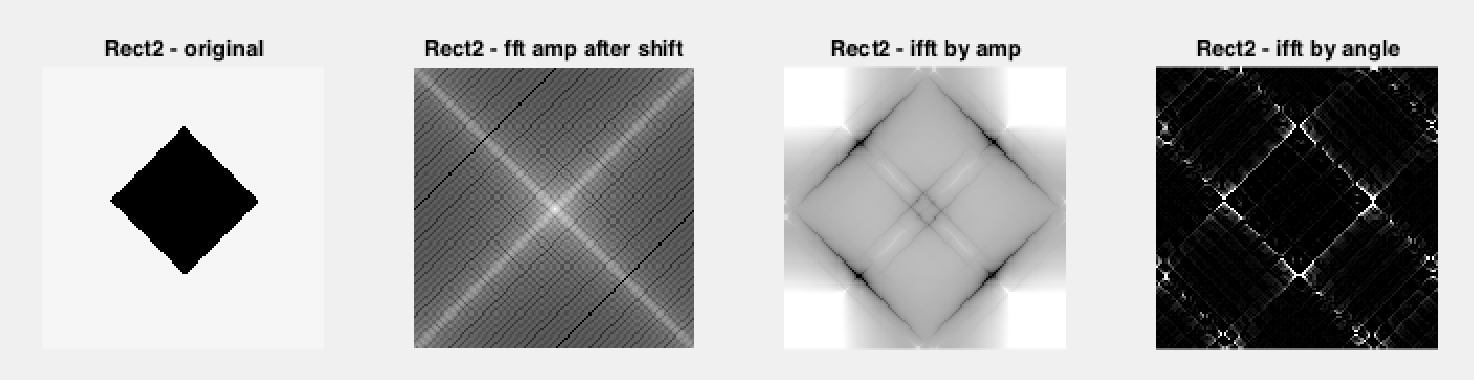我们可以看到幅度FFT正变换和相位FFT你变换都是旋转了45度，但是幅度FFT逆变换区别较大。


展开全文图像处理 matlab 傅立叶变换 图像傅立叶变换
• 实现图像FFT变换并进行滤波 环境：vs2017 + OpenCV 3.4.1 实验步骤： （1）将输入图像转换成256×256大小，这样可以进行8次蝶形运算 （2）将图像转换为灰度图像，并显示 （3）设计FFT输入矩阵，该矩阵为2维双通道...
实现图像FFT变换并进行滤波
环境：vs2017 + OpenCV 3.4.1
实验步骤：
（1）将输入图像转换成256×256大小，这样可以进行8次蝶形运算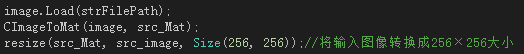（2）将图像转换为灰度图像，并显示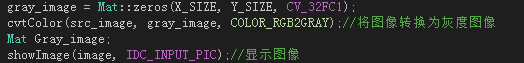（3）设计FFT输入矩阵，该矩阵为2维双通道矩阵，第一个通道为实部，第二个通道为虚部，并将输入矩阵的值赋给实部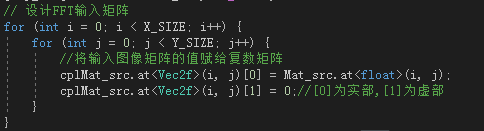（4）将输入矩阵按行进行一维FFT，256个点需进行8次蝶形运算，循环256次得到FFT中间过程矩阵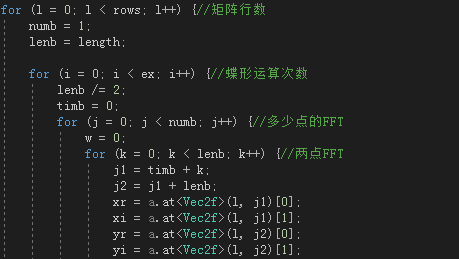（5）矩阵转置，进行列FFT，再次转置，得到FFT频谱矩阵，若要将频谱显示出来，需要求出频谱振幅矩阵，并转换为单通道整型矩阵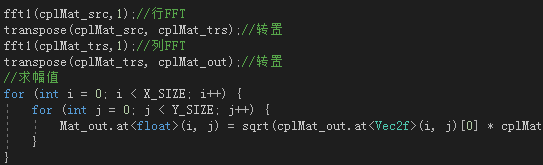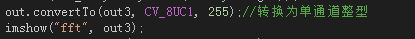（6）带通滤波，滤波范围为60-100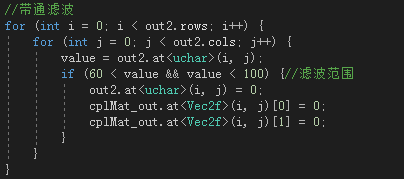（7）将滤波后的矩阵进行IFFT，同样先对行进行FFT，转置后对列进行FFT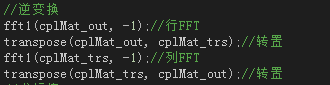（8）求幅值，并转换为单通道整型，并显示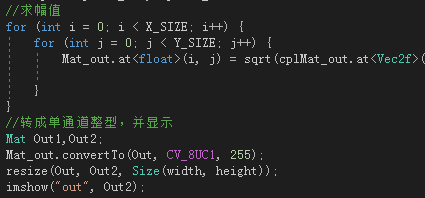实验结果：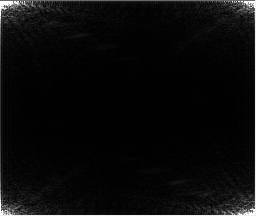FFT频谱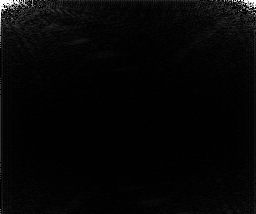滤波后FFT频谱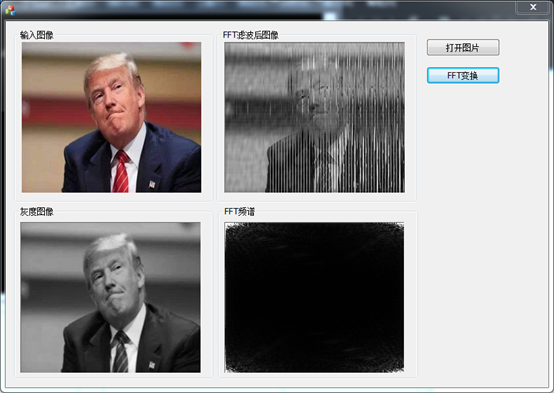核心代码：

void CPPSWDlg::fft1(Mat a,int mode) {//mode=1为FFT，mode=-1为IFFT
int cols = a.cols;
int length = a.cols;
int rows = a.rows;
printf("length=%d\n", cols);
printf("rows=%d\n", rows);
int ex = 0;
int isLing = length;
while (isLing != 0) {
isLing = isLing / 2;
ex += 1;
}
ex = ex - 1;
printf("ex=%d\n", ex);
int bit, kk;
double xx;
xx = -PI * 2.0 / (double)length;
if (mode == 1) {
xx = xx;
}
if (mode == -1) {
xx = -xx;
}

int i, ii, j, k, l, w, j1, j2;
int numb, lenb, timb;
float xr, xi, yr, yi, nrml;
for (l = 0; l < rows; l++) {//矩阵行数
numb = 1;
lenb = length;

for (i = 0; i < ex; i++) {//蝶形运算次数
lenb /= 2;
timb = 0;
for (j = 0; j < numb; j++) {//多少点的FFT
w = 0;
for (k = 0; k < lenb; k++) {//两点FFT
j1 = timb + k;
j2 = j1 + lenb;
xr = a.at<Vec2f>(l, j1);
xi = a.at<Vec2f>(l, j1);
yr = a.at<Vec2f>(l, j2);
yi = a.at<Vec2f>(l, j2);
a.at<Vec2f>(l, j1) = xr + yr;
a.at<Vec2f>(l, j1) = xi + yi;
//printf("cplMat_src.at<Vec2f>(%d, %d)=%f\n", l,j1,cplMat_src.at<Vec2f>(l, j1));
//printf("cplMat_src.at<Vec2f>(%d, %d)=%f\n",l,j1, cplMat_src.at<Vec2f>(l, j1));
xr = xr - yr;
xi = xi - yi;
a.at<Vec2f>(l, j2) = xr * cos(w*xx) - xi * sin(w*xx);
a.at<Vec2f>(l, j2) = xr * sin(w*xx) + xi * cos(w*xx);
//printf("cplMat_src.at<Vec2f>(%d, %d)=%f\n", l,j2,cplMat_src.at<Vec2f>(l, j2));
//printf("cplMat_src.at<Vec2f>(%d, %d)=%f\n",l,j2, cplMat_src.at<Vec2f>(l, j2));
w += numb;
}
timb += (2 * lenb);
}
numb *= 2;
}
for (i = 0; i < length; i++) {
for (kk = 0, ii = i, bit = 0; ; bit <<= 1, ii >>= 1) {
bit = (ii & 1) | bit;
if (++kk == ex) break;
}
cplMat_out.at<Vec2f>(l, i) = a.at<Vec2f>(l, bit);
cplMat_out.at<Vec2f>(l, i) = a.at<Vec2f>(l, bit);
}
for (i = 0; i < length; i++) {
a.at<Vec2f>(l, i) = cplMat_out.at<Vec2f>(l, i);
a.at<Vec2f>(l, i) = cplMat_out.at<Vec2f>(l, i);
}
nrml = 1.0 / sqrt((double)length);
//printf("nrml=%f\n", nrml);
for (i = 0; i < length; i++) {
a.at<Vec2f>(l, i) *= nrml;
a.at<Vec2f>(l, i) *= nrml;
//printf("cplMat_src.at<Vec2f>(%d, %d)=%f\n", l, i, a.at<Vec2f>(l, i));
//printf("cplMat_src.at<Vec2f>(%d, %d)=%f\n", l, i, a.at<Vec2f>(l, i));
}
}
}




展开全文数字图像处理 OpenCV FFT变换 带通滤波
• 希望大伙喜欢！学数字图像处理的基础！matlab源程序
• 一个很实用的图像变换处理，利用labview实现图像的傅里叶FFT变换
• **傅里叶变换： X(f)=∫∞−∞x(t)e−i2πftdtX(f)=\int_{-\infty}^{\infty}x(t)e^{-i2\pi ft} dt 傅里叶逆变换： x(t)=∫∞−∞X(f)ei2πftdfx(t)=\int_{-\infty}^{\infty}X(f)e^{i2\pi ft} df X(f)为x(t)的...图像处理 应用
• 学习DIP第7天，图像傅里叶变换 转载请标明出处：http://blog.csdn.net/tonyshengtan，欢迎大家转载，发现博客被某些论坛转载后，图像无法正常显示，无法正常表达本人观点，对此表示很不满意。。。。。。。。   ...傅里叶变换 图像傅里叶谱 二维傅里叶变换
• 采用FFT（快速傅立叶变换）的方法对图像进行变换
• 图像的傅立叶变换 1.启动MATLAB程序，读入一幅图像；对图像FFT。使用’subplot’命令，同时显示原始图像其频谱图； 1.1实验过程： 首先读取一幅图像，然后将这幅图像归一化到0~1之间，然后对图像做...数字图像
• 细节，值得注意的是：二维FFT可以对图像进行变换，先对每一行进行FFT变换，再对变换后的每一列进行FFT变换， 二维FFT变换的公式如下： 2.复数工具类  FFT和二维FFT都需要复数的加减乘除，在这里给java
•  一般FFT在通信等领域都做的一维变换就可以了，但是在图像处理方面，需要做二维变换，这个时候就需要用到FFT2. 在利用Octave(或者matlab)里面的fft2()函数的时候，观察频率领域的图像还是要点额外的技巧的...信号与系统 图像处理
• 之前写过的一个博客，咋那里面有DFT的matlab 代码，这次这个是C语言代码，欢迎下载。
• 离散傅里叶变换(discrete Fourier transform) ...在数字图像处理中，FFT的使用非常普遍，是图像处理中最重要的算法之一。在此，我们对FFT算法做一些简单研究，并使用python实现该算法，同时会对图像进行变换分析。python 图像处理 快速傅里叶变换
• 基于C/C++的FFT变换，已在PC端和ARM端进行验证，支持以下特性： 1. 1维任意点FFT变换及反变换 2. 2维（图像）任意点FFT变换及反变换 3. 2维频谱相乘 4. 图像频域相关卷积计算
• 二维Fourier变换的应用 前面已经提到了Fourier变换有两个好处，即：可以获得信号的频域特性；可以将卷积运算转换为乘积运算。 因此二维Fourier变换的应用也是根据这两个特点来进行的。 在图像滤波中的应用 首先...图像处理 算法
• 本文主要讲解图像傅里叶变换的相关内容，在数字图像处理中，有两个经典的变换被广泛应用——傅里叶变换和霍夫变换。其中，傅里叶变换主要是将时间域上的信号转变为频率域上的信号，用来进行图像除噪、图像增强等处理...Python图像处理 傅里叶变换 OpenCV 傅里叶逆变换
• 实验内容1.1 使用平台及语言1.2 代码流程1.3 FFT、IFFT2. 实验结果2.1 输入图片及其频谱2.2 进行低频滤波2.3 去除直流分量2.4 低频滤波2.5 高频滤波2.6 进一步的高频率波2.7 更进一步的高频滤波3. 遇到的问题及收获...
• 图像的傅立叶变换可以参考这里：数字图像处理与Python实现-图像变换-傅立叶变换 2. 傅立叶卷积性质数学表示 如果f(x)f(x)f(x)和g(x)g(x)g(x)为两个一维时域函数；f(x,y)f(x,y)f(x,y)和g(x,y)g(x,y)g(x,y)为两个二维...图像处理 快速傅立叶变换 图像卷积 计算机视觉 人工智能
• 傅立叶变换图像处理中有非常非常的作用。因为不仅傅立叶分析涉及图像处理的很多方面，傅立叶的改进算法， 比如离散余弦变换，gabor与小波在图像处理中也有重要的分量。 印象中，傅立叶变换图像处理以下几...
...